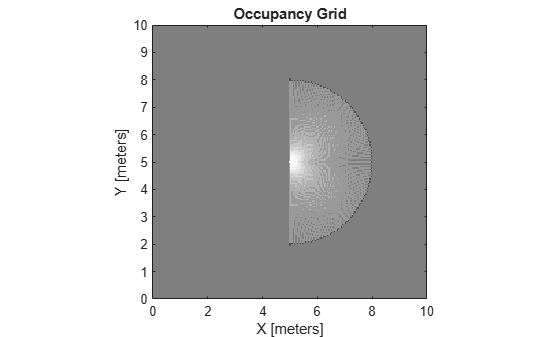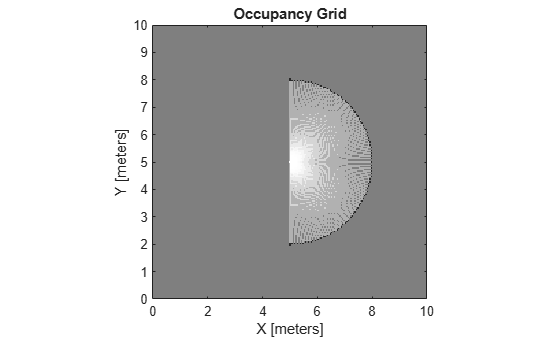insertRay

Insert ray from laser scan observation

Description

example

insertRay(map,pose,scan,maxrange) inserts one or more lidar scan sensor observations in the occupancy grid, map, using the input lidarScan object, scan, to get ray endpoints. The ray endpoints are considered free space if the input scan ranges are below maxrange. Cells observed as occupied are updated with an observation of 0.7. All other points along the ray are treated as obstacle free and updated with an observation of 0.4. Endpoints above maxrange are not updated. NaN values are ignored. This behavior correlates to the inverse sensor model.

insertRay(map,pose,ranges,angles,maxrange) specifies the range readings as vectors defined by the input ranges and angles.

insertRay(map,startpt,endpoints) inserts observations between the line segments from the start point to the end points. The endpoints are updated with a probability observation of 0.7. Cells along the line segments are updated with an observation of 0.4.

insertRay(___,invModel) inserts rays with updated probabilities given in the two-element vector, invModel, that corresponds to obstacle-free and occupied observations. Use any of the previous syntaxes to input the rays.

Examples

collapse all

Create an empty occupancy grid map.

map = occupancyMap(10,10,20);

Input pose of the vehicle, ranges, angles, and the maximum range of the laser scan.

pose = [5,5,0];
ranges = 3*ones(100,1);
angles = linspace(-pi/2,pi/2,100);
maxrange = 20;

Create a lidarScan object with the specified ranges and angles.

scan = lidarScan(ranges,angles);

Insert the laser scan data into the occupancy map.

insertRay(map,pose,scan,maxrange);

Show the map to see the results of inserting the laser scan.

show(map)Check the occupancy of the spot directly in front of the vehicle.

getOccupancy(map,[8 5])
ans = 0.7000

Add a second reading and view the update to the occupancy values. The additional reading increases the confidence in the readings. The free and occupied values become more distinct.

insertRay(map,pose,scan,maxrange);
show(map)getOccupancy(map,[8 5])
ans = 0.8448

Input Arguments

collapse all

Map representation, specified as a occupancyMap object. This object represents the environment of the vehicle. The object contains a matrix grid with values representing the probability of the occupancy of that cell. Values close to 1 represent a high probability that the cell contains an obstacle. Values close to 0 represent a high probability that the cell is not occupied and obstacle free.

Position and orientation of vehicle, specified as an [x y theta] vector. The vehicle pose is an x and y position with angular orientation theta (in radians) measured from the x-axis.

Lidar scan readings, specified as a lidarScan object.

Range values from scan data, specified as a vector of elements measured in meters. These range values are distances from a sensor at given angles. The vector must be the same length as the corresponding angles vector.

Angle values from scan data, specified as a vector of elements measured in radians. These angle values correspond to the given ranges. The vector must be the same length as the corresponding ranges vector.

Maximum range of laser range sensor, specified as a scalar in meters. Range values greater than or equal to maxrange are considered free along the whole length of the ray, up to maxrange.

Start point for rays, specified as a two-element vector, [x y], in the world coordinate frame. All rays are line segments that originate at this point.

Endpoints for rays, specified as an n-by-2 matrix of [x y] pairs in the world coordinate frame, where n is the length of ranges or angles. All rays are line segments that originate at startpt.

Inverse sensor model values, specified as a two-element vector corresponding to obstacle-free and occupied probabilities. Points along the ray are updated according to the inverse sensor model and the specified range readings. NaN range values are ignored. Range values greater than maxrange are not updated. See Inverse Sensor Model.

collapse all

Inverse Sensor Model

The inverse sensor model determines how values are set along a ray from a range sensor reading to the obstacles in the map. You can customize this model by specifying different probabilities for free and occupied locations in the invModel argument. NaN range values are ignored. Range values greater than maxrange are not updated.Grid locations that contain range readings are updated with the occupied probability. Locations before the reading are updated with the free probability. All locations after the reading are not updated.